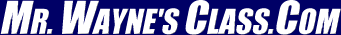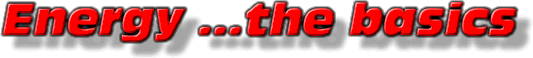This text is meant to accompany class discussions. It is not everything there is to know about energy. It is meant as a  prep for class. More detailed notes and examples are given in the class notes, presentations, and demonstrations linked below under unit resources.

Units Resources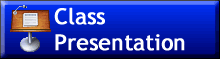(01) Introduction to energy in a "Perfect World" Defines kinetic energy, rest potential energy (e=mc2,) gravitationalpotnetial eergy, Solving with ratios.(02) Application of the conservation of mechanical energy, example problems.(03) Work as a transfer of energy(04) Power(05) Some answers to problems from the workbook.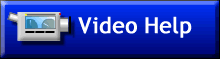Example problems and more.This is the worksheet that goes with the activity above. It is now done as a GoogleDoc."The Jerk" roller coaster worksheet.Flash cards you can use to help you memorize the facts.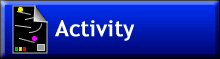This is the introductory inquiry activity. It is a conceptual look at energy, height and speed. (Click here for the html 5 version)This a lab where you investigate the coefficient of restitution. This is now done on GoogleDocs. This is the online version.This is the student workbook containing a nearly all of problems that I use. It is in 4 or more pdf files.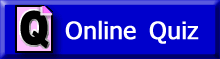Online quiz number 1Online quiz number 2Online quiz number 3Online quiz number 4Energy Mastery Assignment 2022
Objectives
Students will be able to:
1. For all equations, write what each variable stands for and its S.I. unit.
2. Identify the correct S.I. units for ALL forms of Energy.
3. Recall the name of the person who the energy unit is named after.
4. Write the equation for kinetic energy.
5. Define kinetic energy.
6. Identify when an object possesses kinetic energy.
7. Calculate the kinetic energy of an object.
8. Write the equation for potential energy due to gravity.
9. Define potential energy.
10. Identify when an object possesses potential energy.
11. Calculate the potential energy of an object.
12. Solve problems based on conservation of mechanical energy. -Neglect friction and normal forces.
13. Correctly identify the S.I. unit of “work.”
14. Define what “work” is.
15. Write the equation for “work.”
16. Calculate “work” when the force and displacement are parallel.
17. Calculate “work” when the force and displacement are not parallel.
18. Identify “work” as being positive or negative.
19. Identify “work” as being done “on” or “by” the system.
20. Calculate the work on a graph of force vs. displacement.
21. Solve problems using energy – work relationships and ratios.
22. Solve problems involving up to all two types of energies and work.
23. Recall the person who the unit of power is named after.
24. Write the two equations for power.
25. Define power 2 ways –in terms of work and in terms of force.
26. Calculate the power delivered or received by an object

Altermnate Approach Order

Introduction

Energy describes the ability to move a body by performing work. "Work" is the term used to describe how energy is exchanged. If energy it given to a football player from another player, then a physicist says one player "does work on" the other player. More about work later.

Energy is conserved. In a closed system, there is "X" amount of energy. A system is a collection of bodies that interact with each other but are isolated from their surrounding environment. A system could also be defined as a single body. Within the system, energy is may change forms. There are many different forms of energy.

by Tony Wayne ...(If you are a teacher, please feel free to use these resources in your teaching.)

The owner of this website does not collect cookies when the site is visited. However, this site uses and or embeds Adobe, Apple, GoDaddy, Google, and YouTube products. These companies collect cookies when their producs are used on my pages. Click here to go to them to find out more about how they use their cookies. If you do not agree with any of their policies then leave this site now.